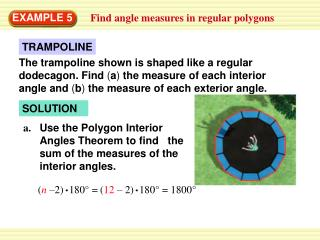DownloadDownload PresentationEXAMPLE 5

# EXAMPLE 5

Download Presentation## EXAMPLE 5

- - - - - - - - - - - - - - - - - - - - - - - - - - - E N D - - - - - - - - - - - - - - - - - - - - - - - - - - -
##### Presentation Transcript

1. The trampoline shown is shaped like a regular dodecagon. Find (a) the measure of each interior angle and (b) the measure of each exterior angle. a. Use the Polygon Interior Angles Theorem to find the sum of the measures of the interior angles. (n –2) 180° = (12 – 2) 180° = EXAMPLE 5 Find angle measures in regular polygons TRAMPOLINE SOLUTION 1800°

2. Then find the measure of one interior angle. A regular dodecagon has 12 congruent interior angles. Divide 1800° by 12: 1800° 12= 150°. ANSWER The measure of each interior angle in the dodecagon is 150°. EXAMPLE 5 Find angle measures in regular polygons

3. By the Polygon Exterior Angles Theorem, the sum of the measures of the exterior angles, one angle at each vertex, is 360°. Divide 360° by 12 to find the measure of one of the 12 congruent exterior angles: 360° 12 = 30°. b. ANSWER The measure of each exterior angle in the dodecagon is 30°. EXAMPLE 5 Find angle measures in regular polygons

4. 6. An interior angle and an adjacent exterior angle of a polygon form a linear pair. How can you use this fact as another method to find the exterior angle measure in Example 5? ANSWER Linear pairs are Supplementary, Since the Interior Angle measures 150°, the exterior angle must measure 30°. for Example 5 GUIDED PRACTICE